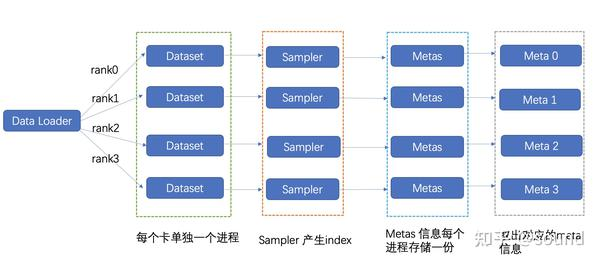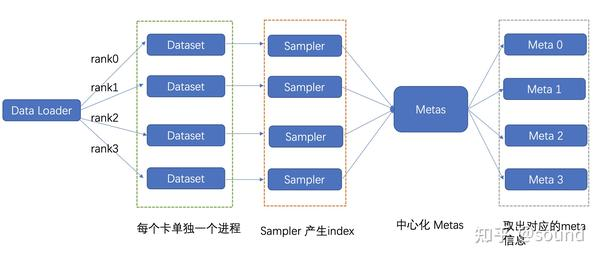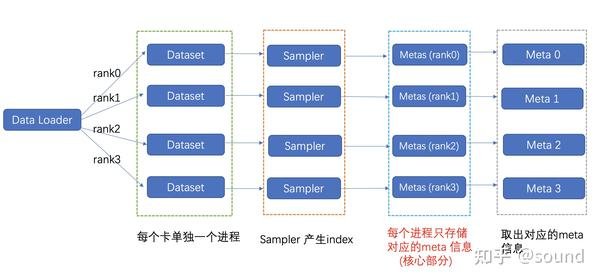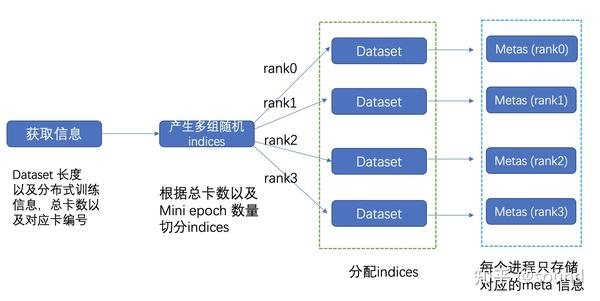# RankDataset：超大规模数据集加载利器

## 问题阐述• meta_file 格式
#filename label
image1.jpg "balabala"
image2.jpg "balabala"
image3.jpg "balabala"
• NaiveDataset
from torch.utils.data import Dataset
from torch.utils.data import DataLoader
from torch.utils.data.sampler import Sampler

class NaiveDataset(Dataset):
def __init__(self, meta_file):
super(NaiveDataset, self).__init__()
self.metas = self.parse(meta_file)

def parse(self, meta_file):
metas = []
with open(meta_file) as f:
for line in f.readlines():
metas.append(line.strip())
return metas

def __getitem__(self, idx):
return self.metas[idx]

def __len__(self):
return len(self.metas)
• RandomSampler
class RandomSampler(Sampler):
r"""Samples elements randomly, without replacement.

Arguments:
data_source (Dataset): dataset to sample from
"""

def __init__(self, dataset):
self.dataset = dataset

def __iter__(self):
return iter(torch.randperm(len(self.dataset)).tolist())

def __len__(self):
return len(self.dataset)

dataset = NaiveDataset("/path/to/meta")
sampler = RandomSampler(datset)
dataloader = DataLoader(
dataset=dataset,
batch_size=32,
shuffle=False,
num_workers=4,
sampler=sampler
)
model = build_model()
for index, batch in enumerate(dataloader):
image, label = batch
output = model(image)
loss = criterion(output, label)
loss.backward()

• DistributedRandomSampler
class DistributedRandomSampler(Sampler):
r"""Samples elements randomly, without replacement.

Arguments:
data_source (Dataset): dataset to sample from
"""

def __init__(self, dataset, rank, world_size):
self.dataset = dataset
self.world_size = world_size
self.rank = rank
self.num_samples = int(math.ceil(len(self.dataset) * 1.0 / self.world_size))

def __iter__(self):
index_list = torch.randperm(len(self.dataset)).tolist()
index_list = padding(len(self.dataset), self.rank, self.world_size) #padding函数保证index_list长度整除rank

return iter(index_list[self.rank * self.num_samples: (self.rank + 1) * self.num_samples])

def __len__(self):
return self.num_samples

sampler = DistributedRandomSampler(dataset)

dataloader = DataLoader(
dataset=dataset,
batch_size=32,
shuffle=False,
num_workers=2,
sampler=sampler
)

### 解决方案一

"那怎么解决呢？"小王问表哥。

"Talk is cheap, show me the code\?"class ServerDataset(Dataset):
def __init__(self, meta_file, server_ip, server_port):
super(ServerDataset, self).__init__()
self.server_ip = server_ip
self.server_port = server_port
self.meta_num = get_meta_num(server_ip, server_port)

def get_meta(self, idx):
meta = requests.get('http://{}:{}/get/{}'.format(self.server_ip, self.server_port, idx), timeout=1000).json()
return meta

def __getitem__(self, idx):
return self.get_meta(idx)

def __len__(self):
return self.meta_num

“这种做法对于qps在1k以下还比较实用, 但是当训练的总batchsize 特别大的时候这种做法会有明显的瓶颈问题，受限于中心化的并发读取上限问题，因此此方法具有一定的局限性。”

### 解决方案二• 切分流程
'''
Metas 切分过程, mini_epoch = 2, world_size = 8

mini_epoch_idx = 0                            mini_epoch_idx = 1
---- ---- ---- ---- ---- ---- ---- ---- | ---- ---- ---- ---- ---- ---- ---- ----
rk0  rk1  rk2  rk3  rk4  rk5  rk6  rk7  | rk0  rk1  rk2  rk3  rk4  rk5  rk6  rk7

'''

• 本地读取样例class RankDataset(Dataset):
'''
实际流程
获取rank和world_size 信息 -> 获取dataset长度 -> 根据dataset长度产生随机indices ->
给不同的rank 分配indices -> 根据这些indices产生metas

'''
def __init__(self, meta_file, world_size, rank, seed):
super(RankDataset, self).__init__()
random.seed(seed)
np.random.seed(seed)
self.world_size = world_size
self.rank = rank

self.metas = self.parse(meta_file)

def parse(self, meta_file):
dataset_size = self.get_dataset_size(meta_file)                                     # 获取metafile的行数
local_rank_index = self.get_local_index(dataset_size, self.rank, self.world_size)   # 根据world size和rank，获取当前epoch，当前rank需要训练的index。
self.metas = self.read_file(meta_file, local_rank_index)

def __getitem__(self, idx):
return self.metas[idx]

def __len__(self):
return len(self.metas)

epoch_num = 0
dataset = RankDataset("/path/to/meta", world_size, rank, seed=epoch_num)
sampler = RandomSampler(datset)
dataloader = DataLoader(
dataset=dataset,
batch_size=32,
shuffle=False,
num_workers=4,
sampler=sampler
)

for epoch_num in range(epoch_num):

dataset = RankDataset("/path/to/meta", world_size, rank, seed=epoch_num)
sampler = RandomSampler(datset)
dataloader = DataLoader(
dataset=dataset,
batch_size=32,
shuffle=False,
num_workers=4,
sampler=sampler
)

## 总结

• 自己实现一个继承torch.data.Dataset类就可以，需要实现init,getitem,len三个函数；
• 使用torch默认的RandomSampler即可满足一般的random shuffle需求
• 使用torch默认的dataloader就制定完成数据迭代器

• Dataset保持不变
• sampler进行修改，保证每个rank读到的index可以覆盖到整个dataset，并且每个rank读的数据要是等量的
• dataloader保持不变

• Dataset进行修改， getitem从内存读取数据改成向server发出请求，获得对应index的数据。
• 可以直接使用分布式的sampler
• dataloader保持不变

RankDataset：

• 支持进一步切分数据集，分批去读取数据集。
• Dataset进行修改：每个epoch先计算该rank需要使用的index，然后根据index获取meta_file对应行，加载到内存中。
• 改为torch默认的使用torch默认的RandomSampler即可满足一般的random。
• dataloader保持不变，但是在训练过程中，每个epoch到要用不同的随机数重新build dataloader。

## 后记

RankDataset已经在公司内部的分类和检测框架（POD）进行精度和速度验证，同时已经集成到Spring2 内部，方便公司内部用户的使用，欢迎各位使用。